# 【论文阅读】Batch Normalization：Accelerating Deep Network Training by Reducing Internal Covariate Shift

## Batch Normalization，BN-Inception

Posted by x-jeff on November 2, 2021

# 3.Normalization via Mini-Batch Statistics

$\hat{x}^{(k)}=\frac{x^{(k)}-E[x^{(k)}]}{\sqrt{Var[x^{(k)}]}}$

$y^{(k)}=\gamma^{(k)} \hat{x}^{(k)} + \beta^{(k)}$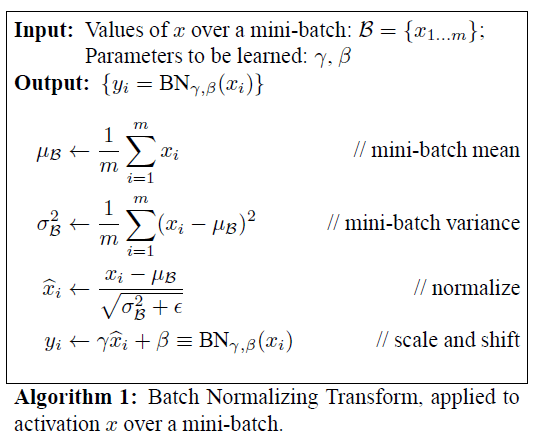$\frac{\partial \ell}{\partial \hat{x}_i}=\frac{\partial \ell}{\partial y_i} \cdot \gamma$ $\frac{\partial \ell}{\partial \sigma^2_\mathcal{B}} = \sum^m_{i=1} \frac{\partial \ell}{\partial \hat{x}_i} \cdot (x_i - \mu_{\mathcal{B}})\cdot \frac{-1}{2}(\sigma^2_{\mathcal{B}}+\epsilon)^{-3/2}$ $\frac{\partial \ell}{\partial \mu_{\mathcal{B}}}=\left( \sum^m_{i=1} \frac{\partial \ell}{\partial \hat{x}_i} \cdot \frac{-1}{\sqrt{\sigma^2_{\mathcal{B}}+\epsilon}} \right)+\frac{\partial \ell}{\partial \sigma^2_{\mathcal{B}}} \cdot \frac{\sum^m_{i=1}-2(x_i-\mu_{\mathcal{B}})}{m}$ $\frac{\partial \ell}{\partial x_i}=\frac{\partial \ell}{\partial \hat{x}_i} \cdot \frac{1}{\sqrt{\sigma^2_{\mathcal{B}}+\epsilon}}+\frac{\partial \ell}{\partial \sigma^2_{\mathcal{B}}} \cdot \frac{2(x_i-\mu_{\mathcal{B}})}{m} + \frac{\partial \ell}{\partial \mu_{\mathcal{B}}}\cdot \frac{1}{m}$ $\frac{\partial \ell}{\partial \gamma}=\sum^m_{i=1} \frac{\partial \ell}{\partial y_i} \cdot \hat{x}_i$ $\frac{\partial \ell}{\partial \beta}=\sum^m_{i=1} \frac{\partial \ell}{\partial y_i}$

## 3.1.Training and Inference with Batch-Normalized Networks

$Var[x]=\frac{m}{m-1} \cdot E_{\mathcal{B}}[\sigma^2_{\mathcal{B}}]$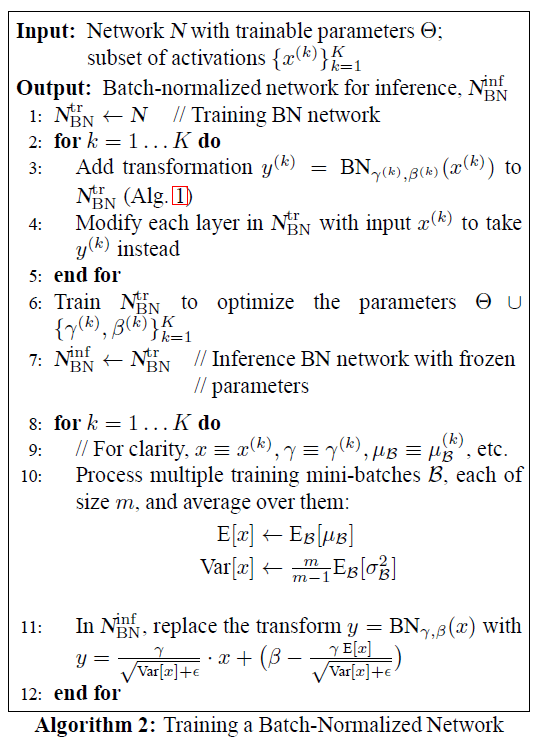k指的是网络的第k层，一共为K层。

## 3.2.Batch-Normalized Convolutional Networks

BN可以应用于网络的任何部分。我们只考虑对激活函数的输入$x$进行BN：

$z=g(Wu+b)$ $x=Wu+b$

$g(\cdot)$为非线性激活函数，例如ReLU。如果选择对$u$进行BN（$u$其实就是激活函数的输出），其分布形状在训练期间可能会改变，从而影响对covariate shift的消除。相比较而言，$x$的分布更具对称性和非稀疏性。

$z=g(BN(Wu))$

# 4.Experiments

## 4.1.Activations over time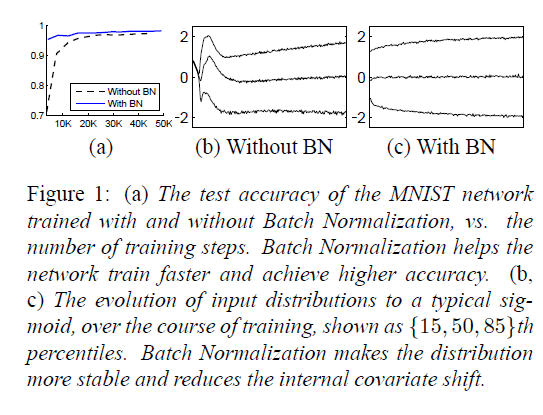## 4.2.ImageNet classification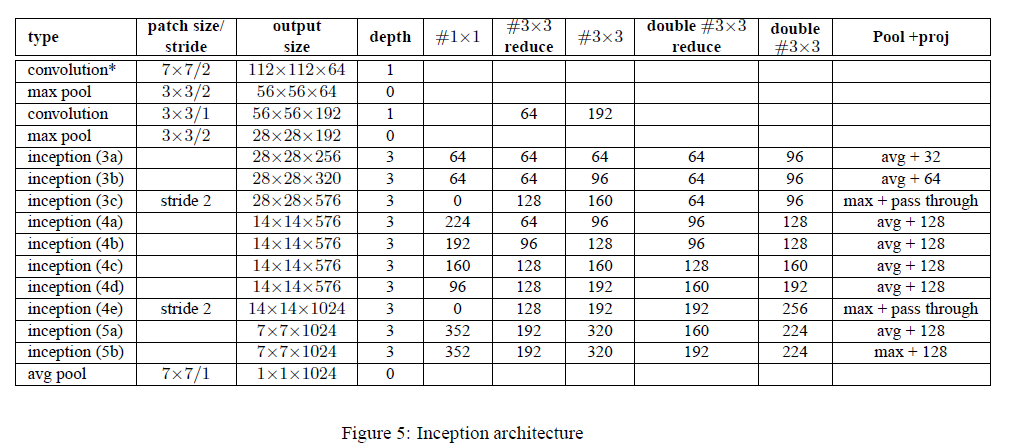1. 将Inception模块中的$5\times 5$卷积层改成了两个连续的$3\times 3$卷积层。所以在Fig(5)中inception模块的深度从2变成了3。
2. 在Fig(5)中，第二个卷积层的深度为1。个人觉得这里作者应该是写错了，因为有“#$3\times 3$ reduce”和“#$3\times 3$”，和GoogLeNet是一样的，所以其深度应为2。
3. 把部分inception模块中的max pool改为了avg pool。
4. 修改了使用的inception模块数量（例如添加了inception3(c)）及输出维度。

### 4.2.1.Accelerating BN Networks

👉Increase learning rate.

👉Remove Dropout.

👉Reduce the L2 weight regularization.

👉Accelerate the learning rate decay.

👉Remove Local Response Normalization.

👉Shuffle training examples more thoroughly.

👉Reduce the photometric distortions.

### 4.2.2.Single-Network Classification

1. Inception：见Fig5。初始学习率为0.0015。
2. BN-Baseline：在1的基础上，每个非线性激活函数前都使用了BN。
3. BN-x5：在2的基础上，添加了第4.2.1部分所提到的修改。其中，初始学习率提升5倍，改为0.0075。
4. BN-x30：和3唯一的不同是初始学习率提升了30倍，为0.045。
5. BN-x5-Sigmoid：和3唯一的不同是把ReLU激活函数换成了sigmoid激活函数。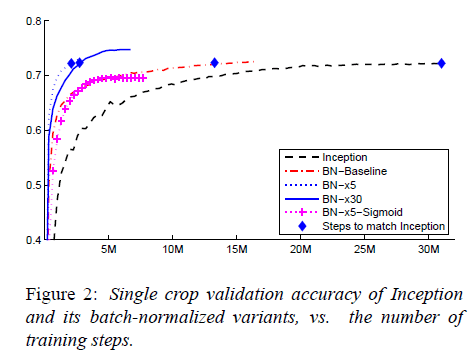Inception在训练了$31\cdot 10^6$步后达到了72.2%的准确率（Inception所能达到的最高准确率）。BN-Baseline达到72.2%准确率所用的步数不到Inception的一半。BN-x5达到72.2%准确率所用步数仅为Inception的十四分之一。BN-x30相比BN-x5训练速度慢了一些，但是最终的准确率更高，其在$6\cdot 10^6$步时达到了74.8%的准确率。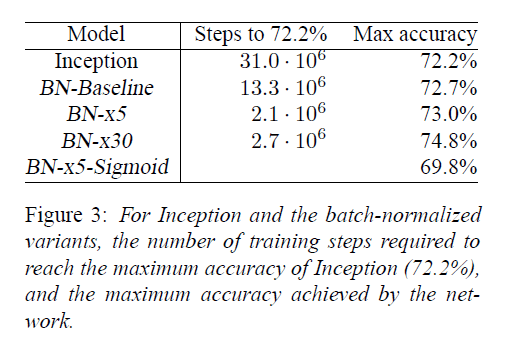### 4.2.3.Ensemble Classification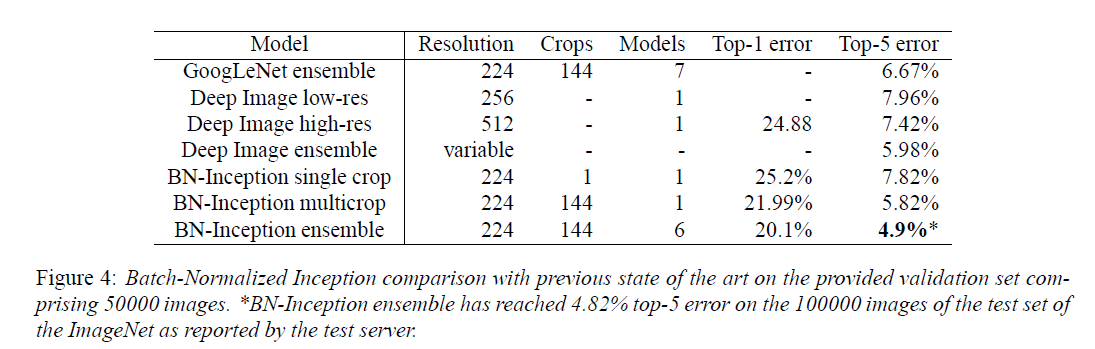BN-Inception ensemble所用的6个模型均基于BN-x30，针对每个模型做了以下修改之一：增大卷积层随机初始化的权值；添加dropout（比例为5%或10%，GoogLeNet中使用的是40%）；对模型的最后一个隐藏层中的每一个神经元进行BN处理（个人理解：即每个神经元都有一组$\gamma,\beta$，不再是一个feature map共用一个）。

# 5.Conclusion

BN可以显著的提升深度神经网络的训练速度。并且在此基础上做一些简单的修改，我们可能会获得更好的结果。多个BN模型的集成同样也有助于获得更好的结果。

# 6.原文链接